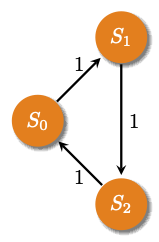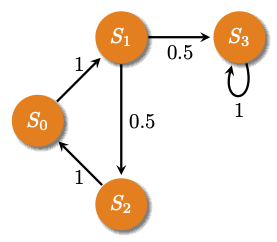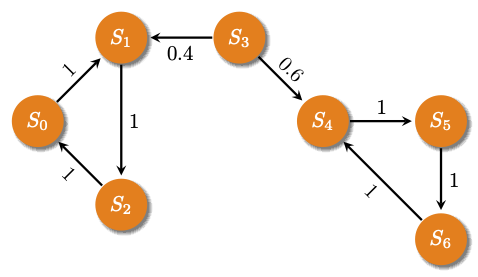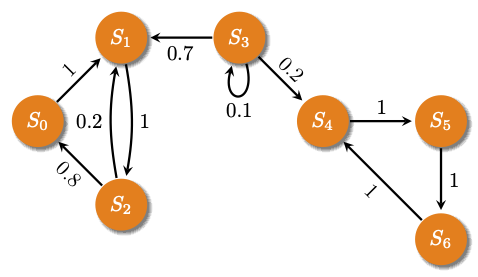# Exercise: Probabilities on small graphs¶

Consider random walks on the following small graphs with the indicated probabilities and answer the questions posed.

Task 1:What is the probability that you can hit one state from another state (in any number of steps) in this Markov chain? Answer by looking at the graph and double check that an eigenvector gives what is expected.

Task 2:For each question below, guess the answer from the figure, and then verify that your computational method gives the expected answer.

• Starting from $B = \{3\}$, what is the probability of hitting $A=\{0, 1, 2\}$ in any number of steps?

• Starting from from any state in $B= \{ 0, 1, 2\}$ what is the probability of hitting $A = \{ 3\}$ in any number of steps?

• Is this an absorbing Markov chain? Verify that the answers come out the same using the two methods you learnt.• What are the hitting probabilities of $A=\{0, 1, 2\}$ from the remaining states?

• What are the hitting probabilities of $A = \{ 4, 5, 6\}$ from the remaining states?

• What are the hitting probabilities of $A = \{3\}$ from the remaining states?